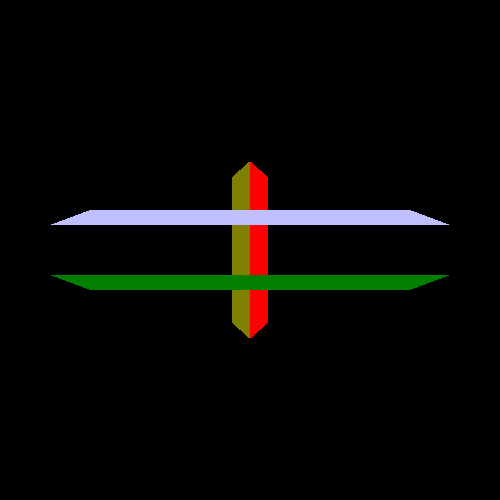## Boundaries and Clipping

If you recall back to the Perspective projection tutorial, we choose to use some special hardware in the graphics chip to do the final division of the W coordinate, rather than doing the entire perspective projection ourselves in the vertex shader. At the time, it was promised that we would see why this is hardware functionality rather than something the shader does.

Let us review the full math operation we are computing here:

Equation 5.1. Perspective Computation

$\stackrel{⇀}{R}=\stackrel{⇀}{P}\left(\frac{{E}_{z}}{{P}_{z}}\right)$

R is the perspective projected position, P is the camera-space position, Ez is the Z-position of the eye relative to the plane (assumed to be -1), and Pz is the camera-space Z position.

One question you should always ask when dealing with equations is this: can it divide by zero? And this equation certainly can; if the camera-space position of any vertex is ever exactly 0, then we have a problem.

This is where clip-space comes in to save the day. See, until we actually do the divide, everything is fine. A 4-dimensional vector that will be divided by the fourth component but has not yet is still valid, even if the fourth component is zero. This kind of coordinate system is called a homogeneous coordinate system. It is a way of talking about things that you could not talk about in a normal, 3D coordinate system. Like dividing by zero, which in visual terms refers to coordinates at infinity.

This is all nice theory, but we still know that the clip-space positions need to be divided by their W coordinate. So how to we get around this problem?

First, we know that a W of zero means that the camera-space Z position of the point was zero as well. We also know that this point must lie outside of the viable region for camera space. That is because of the camera Z range: camera zNear must be strictly greater than zero. Thus any point with a camera Z value of 0 must be in front of the zNear, and therefore outside of the visible world.

Since the vertex coordinate is not going to be visible anyway, why bother drawing it and dividing by that pesky 0? Well, because that vertex happens to be part of a triangle, and if part of the triangle is visible, we have to draw it.

But we do not have to draw all of it.

Clipping is the process of taking a triangle and breaking it up into smaller triangles, such that only the part of the original triangle that is within the viewable region remains. This may generate only one triangle, or it may generate multiple triangles.

Any vertex attributes associated with that vertex are interpolated (based on the vertex shader's interpolation qualifiers) to determine the relative value of the post-clipping vertex.

As you might have guessed, clipping happens in clip space, not NDC space. Hence the name. Since clip-space is a homogeneous coordinate system, we do not have to worry about those pesky zeros. Unfortunately, because homogeneous spaces are not easy to draw, we cannot show you what it would look like. But we can show you what it would look like if you clipped in camera space, in 2D:

Figure 5.6. Triangle ClippingTo see the results of clipping in action, run the Vertex Clipping tutorial. It is the same as the one for depth buffering, except one object has been moved very close to the zNear plane. Close enough that part of it is beyond the zNear and therefore is not part of the viewable area:

Figure 5.7. Near Plane ClippingFork me on GitHub